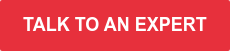# Set the right PID tuning parameters

Set the right PID tuning parameters to achieve optimal plant performance

What do these 3 letters mean and how can they influence your plant behavior?

PID is an acronym for Proportional, Integral, and Derivative. These parameters can be used, adjusted, and controlled individually and collectively. You can have, for example, a P controller, a PI controller, or a PID controller. Each parameter can be adjusted and controlled individually and each parameter can be used for specific purposes.

## What are PID tuning parameters?

The general PID loop consists of three terms:

1. A proportional action on the error or the PV
2. An integral action on the error
3. A derivative action on the error signal or PV

Picking the right equation and choosing the best parameter value is the essence of achieving optimal tuning.

## Description of the PID parameters

What is the role of P, I and D in a PID controller? Understanding the roles is important while tuning.

### Proportional (P) parameter

The P-action is proportional to the error or the PV. The error (or PV) is multiplied with the proportional gain and added to the controller output. The P-action gives the output a ‘kick’ in the right direction.

If the error value is zero, then the P action is zero. This implies that a controller with only P action needs a non-zero error to have a non-zero output. Accurate tracking is therefore not possible with only P control.

### Integral (I) parameter

Consider a plot of the error between PV and SP over time. In mathematics, the “integral” of the error can be interpreted as the surface between the curve and the x-axis and between the y-axis and the current time instant.  Every time step, the plot extends a bit to the right. If the error is zero at that time, the surface does not increase and the integral remains constant.

If your error is positive the surface below the error curve will increase, resulting in a higher controller output. The I action will decrease when the error becomes negative.

Typically the I-action will act much slower compared to the proportional action. However, it will bring the error to zero eventually, which the proportional action can’t do. So basically, the integral action looks at the past and checks if the error is getting to the setpoint. If not, it’s acting on the output. It is going to steer the wheel until you are heading in the intended direction.

### Derivative (D) parameter

The integral doesn’t have the possibility to predict the behavior of error. The derivative action addresses this problem by anticipating the future behavior of the error.

So, the derivative action is the change of the error. It adds a contribution to the output according to how the error changes. When the error is positive, but is starting to decline the D action, it will reduce the output of the controller. It’s the brake that tries to avoid overshoot. It reduces the oscillations induced by the other two actions. It can speed up the controller to the setpoint that we want to achieve.

It reduces the oscillations induced by the other two actions. It can speed up the controller to the setpoint that you want to achieve. However, the derivative action is not often used in PID tuning. The problem is that it can amplify noise. If the error signal is very noisy, the controller output tends to oscillate a lot. This can negatively affect the lifetime of the equipment like pumps and valves.

## Discover if you’re a true PID tuning Master or if you’re still exploring the best PID tune practices.

• Is it a constant challenge to tune your plant’s PID-controllers?
• Are you struggling to get the parameters right the first time, without causing oscillation or overshoot?

Talk to one of our control experts, so we can help you overcome these challenges.